# C Examples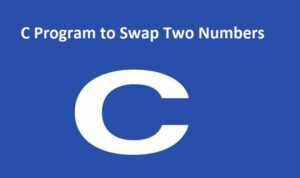#### C Program to Swap Two Numbers

C Program to Swap Two Numbers In this example, you will learn to swap two numbers in C programming using ...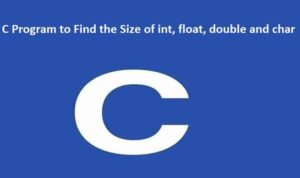#### C Program to Find the Size of int, float, double and char

C Program to Find the Size of int, float, double and char In this example, you will learn to evaluate ...#### C Program to Compute Quotient and Remainder

C Program to Compute Quotient and Remainder In this example, you will learn to discover the quotient and remainder when ...#### C Program to Find ASCII Value of a Character

In this example, you will learn how to find the ASCII value of a character. To understand this example, you ...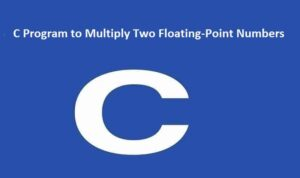#### C Program to Multiply Two Floating-Point Numbers

C Program to Multiply Two Floating-Point Numbers In this example, the product of two floating-point numbers entered by the user ...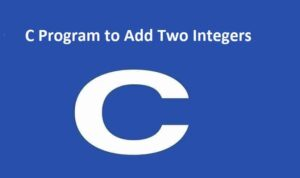#### C Program to Add Two Integers

C Program to Add Two Integers: In this example, the user is asked to enter two integers. Then, the sum ...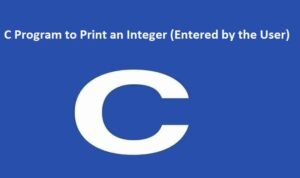#### C Program to Print an Integer (Entered by the User)

C Program to Print an Integer (Entered by the User) In this example, the integer entered by the user is ...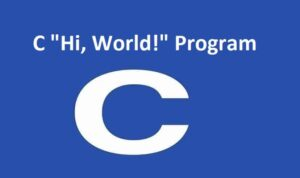#### C “Hello, World!” Program

C "Hello, World!" Program: In this example, you will learn how to print "Hello, World!" on the screen in C ...